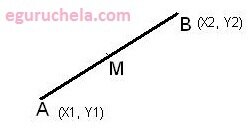# Midpoint Calculator

Calculate the midpoint between two Entered coordinates (x1 , y1 ) and (x2 , y2) in the XY plane by averaging the XY coordinates.

 X1: Y1: X2: Y3:

 Mid Point

The Midpoint Between (x1 , y1 ) and (x2 , y2) points measure a linear midpoint between two locations.

## Midpoint Formula

M = ((x1 + x2)/2 , (y1 + y2)/2)Find the point which is exactly halfway between two given points by averaging the x-values and the y-values. In geometry, the point on a line segment that divides the line into two equal parts.

Therefore we can define the Midpoint as follows:

The line segment AB is a part of the line that is bound by two distinct points A and B, which are called the endpoints of the line segment AB.

The point M is the midpoint of the line segment AB if it is an element of the segment and divides it into two congruent segments, AM and MB. Each segment between the midpoint M and an endpoint have the equal length.

The midpoint is the center, or middle, of a line segment. Any line segment has a unique midpoint.

So, we can find the midpoint of any segment on the coordinate plane by using the mipoint formula.

$$M(x_M,y_M)\equiv M(\frac{x_A + x_B}{2}, \frac{y_A + y_B}{2})$$ $$or\equiv (\frac{x_1 + x_2}{2}, \frac{y_1 + y_2}{2})$$